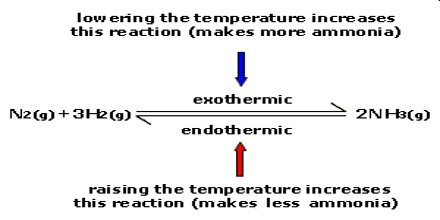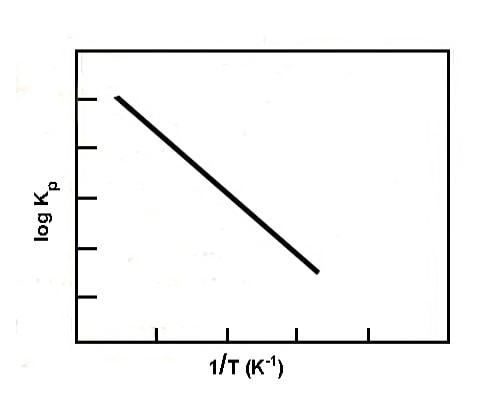# Effect of Temperature on Equilibrium

Effect of temperature on equilibrium

The effect of temperature on equilibrium depends on whether the reaction is exothermic or endothermic. A temperature change occurs when the temperature is increased or decreased by the flow of heat.

According to the principle of Le Chatelier, an increase of temperature will cause the equilibrium to move in the direction in which the effect of the temperature rise may be minimized. For this to happen the equilibrium moves in a direction in which heat is absorbed. In the case of an exothermic reaction, equilibrium moves to the reactant side as in this direction the reaction is endothermic. In the case of an endothermic reaction, equilibrium moves to the product side as the added heat can be used up if more products are formed. Conversely, a decrease of temperature will shift an exothermic equilibrium to the right and an endothermic equilibrium to the left.

These conclusions are illustrated below:

The synthesis of ammonia by the Haber-Bosch process is exothermic

N2 (g) + 3H2 (g) ↔ 2NH3 (g)        ∆H = – 92 kJAs heat is evolved in this reaction an increase of temperature will cause the equilibrium to shift to the left, i.e., the yield of ammonia will he decreased on increasing the temperature. Conversely, if the temperature is decreased more of the product will be formed and the equilibrium shifts to the right.

If a reaction is endodermic, as in the production of nitric oxide by the Birklende and Eide process;

N2 (g) + O2 (g) ↔ 2 NO (g)     ∆H = + 180.0 kJ

an increase in temperature (addition of heat) increases the amount of the product while cooling (removal of heat)  decreases the amount of product, i.e., shifts the equilibrium towards the left.

The change of equilibrium constant with temperature may be expressed quantitatively in the form of the equation;

log KP = ∆H / 2.303 RT + ConstantFig: plot of log Kp vs 1/T (endothermic reaction)

where ∆H is the enthalpy change of the reaction. If ∆His constant, a plot of log Kp vs 1/T gives a straight line whose slope is (∆H / 2.303 R). A schematic representative plot is given in the figure. If ∆H is positive the slope is negative, while if ∆H is negative the slope is positive.

Hence for an exothermic reaction, KP decreases with the increase of temperature, while for an endothermic reaction it increases with a rise in temperature. This is in agreement with Le Chatelier principle.